changing font causes spacing issues for legend #73

Closed
opened this issue Aug 28, 2017 · 15 comments

Projects
None yet

steveharoz commented Aug 28, 2017

 Test case: ``````library(extrafont) #font_import() #<-- run once, takes a long time loadfonts(device="win", quiet=TRUE) plotTemp = ggplot(mtcars) + aes(x=cyl, fill=paste(cyl, "cylinders")) + geom_bar() + theme(text = element_text(family="Arial")) # pick a font on your system `````` The basic plot works fine: ``````plotTemp ``````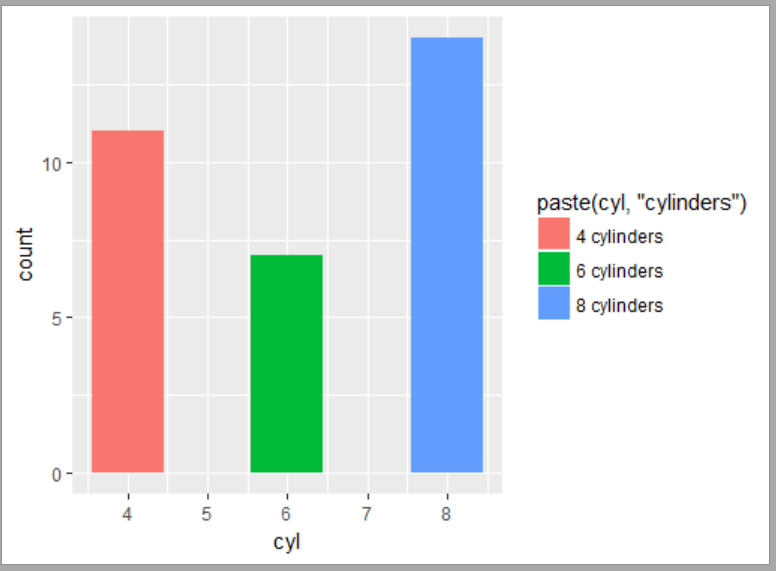Using `plot_grid` clips the legend ``````cowplot::plot_grid(plotTemp, plotTemp, ncol=1, align="v") ``````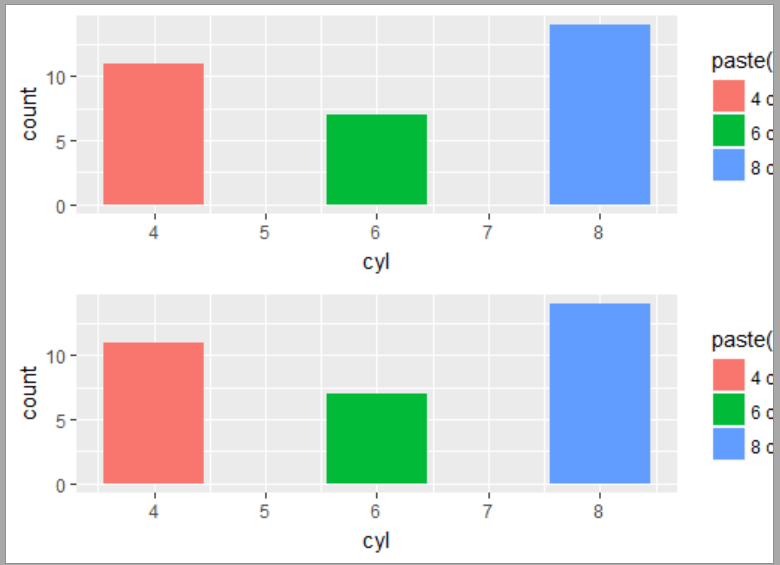Also, there's a weird issue when legend.position = "top" ``````plotTemp = plotTemp + theme(legend.position = "top") cowplot::plot_grid(plotTemp, plotTemp, ncol=1, align="v") ``````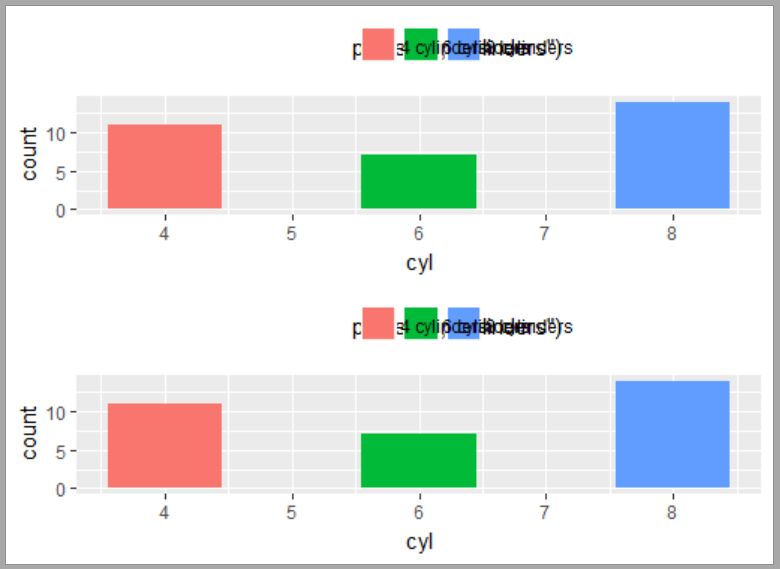Contributor

clauswilke commented Aug 29, 2017

 I doubt the problem is cowplot. Can you try the following: ``````g <- ggplot2::ggplotGrob(plotTemp) grid::grid.draw(g) `````` and see if either of the issues (cut-off or mangled legends) occur? I suspect they will.
Author

steveharoz commented Aug 29, 2017 • edited

 That works fine with the legend in either location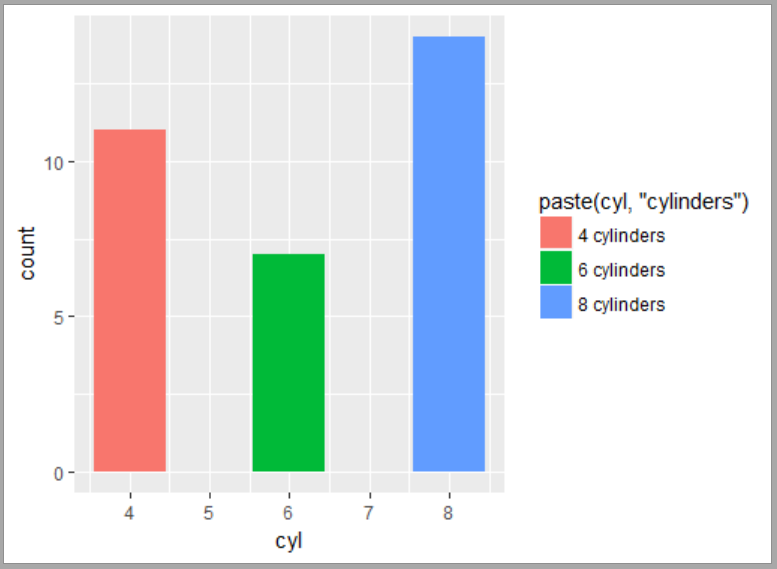Contributor

clauswilke commented Aug 29, 2017

 Ok. Different test: In `plot_grid`, does it break even if you don't use `align = "v"`?
Author

steveharoz commented Aug 29, 2017

 Yeah, that breaks ``````cowplot::plot_grid(plotTemp, plotTemp, ncol=1) ``````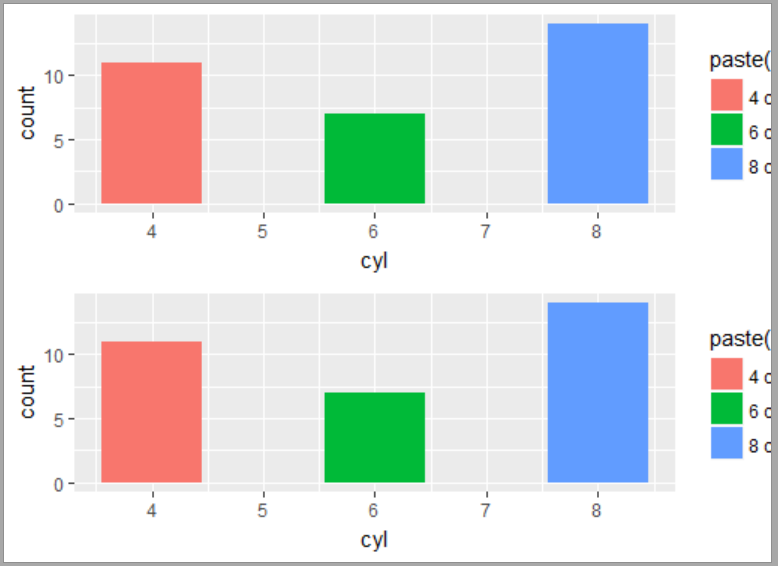Contributor

clauswilke commented Aug 29, 2017

 Another question: How do you generate these figures? R graphics sometimes are cut off when shown in a window, until you resize the window. I'd prefer to talk about code that saves a figure as pdf or png, since that's more reproducible.
Author

steveharoz commented Aug 29, 2017 • edited

 I originally encountered the problem when saving to a file via `CairoPNG`. The screenshots in this thread were from the RStudio plot popout. However, the issue still happens when saving to a file via `ggsave()` with default settings.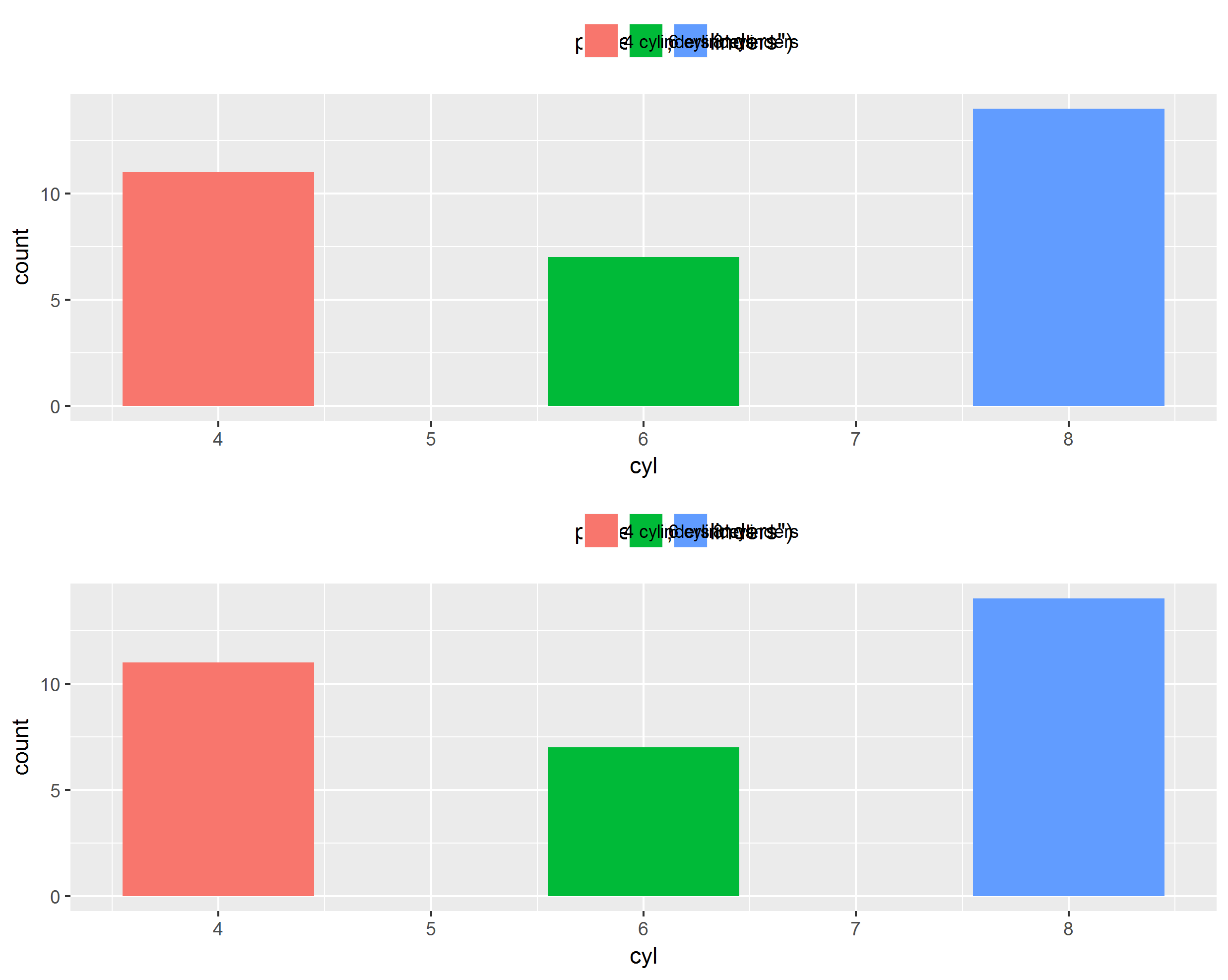Contributor

clauswilke commented Aug 29, 2017

 Ok, this will require some further digging. I can't look into this now but may get back to it at some point.

luizaantunes commented Mar 9, 2018

 Same issue, used: family = "Trebuchet MS" on plot axis and tittles. Tried to fix by adding device=cairo_pdf in ggsave(), it corrected ggsave() but made the cowplot plot_grid() even worse.
Contributor

clauswilke commented Mar 16, 2018

 I can't debug this on windows, because I don't have windows, but from reading the documentation I suspect that `loadfonts(device="win", quiet=TRUE)` may be the culprit. cowplot always uses a pdf device at some point, even if the final output is not pdf. So to use additional fonts, you will have to register the fonts with the pdf device as well.
Contributor

clauswilke commented Mar 16, 2018

 I committed a fix. Can you check it? The following code works for me. `library(extrafont)` should not be needed, but you do need Cairo. ``````library(Cairo) library(cowplot) plotTemp = ggplot(mtcars) + aes(x=cyl, fill=paste(cyl, "cylinders")) + geom_bar() + theme(text = element_text(family="Trebuchet MS")) # pick a font on your system cowplot::plot_grid(plotTemp, plotTemp, ncol=1, align="v") ``````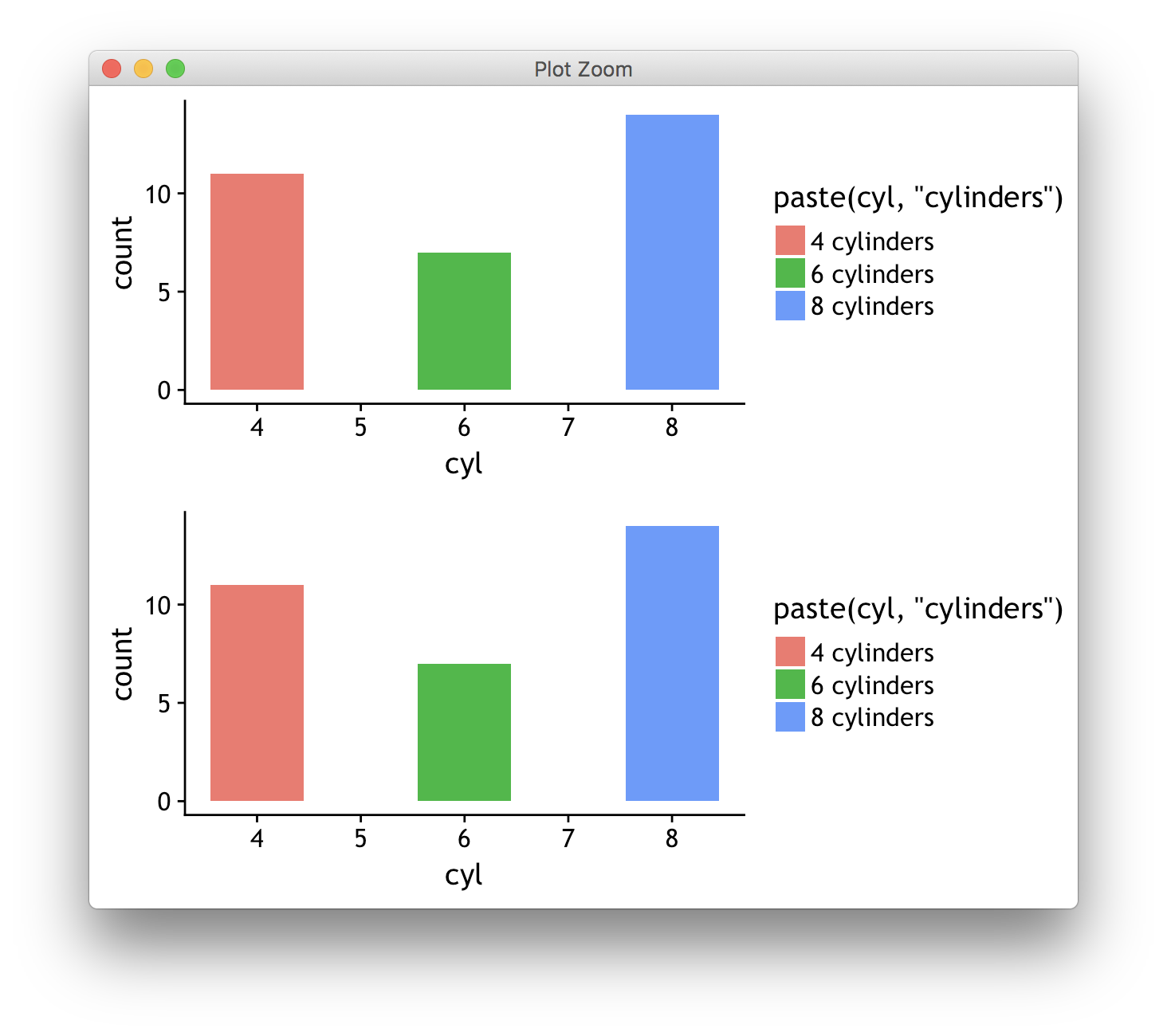``````plotTemp = plotTemp + theme(legend.position = "top") cowplot::plot_grid(plotTemp, plotTemp, ncol=1, align="v") ``````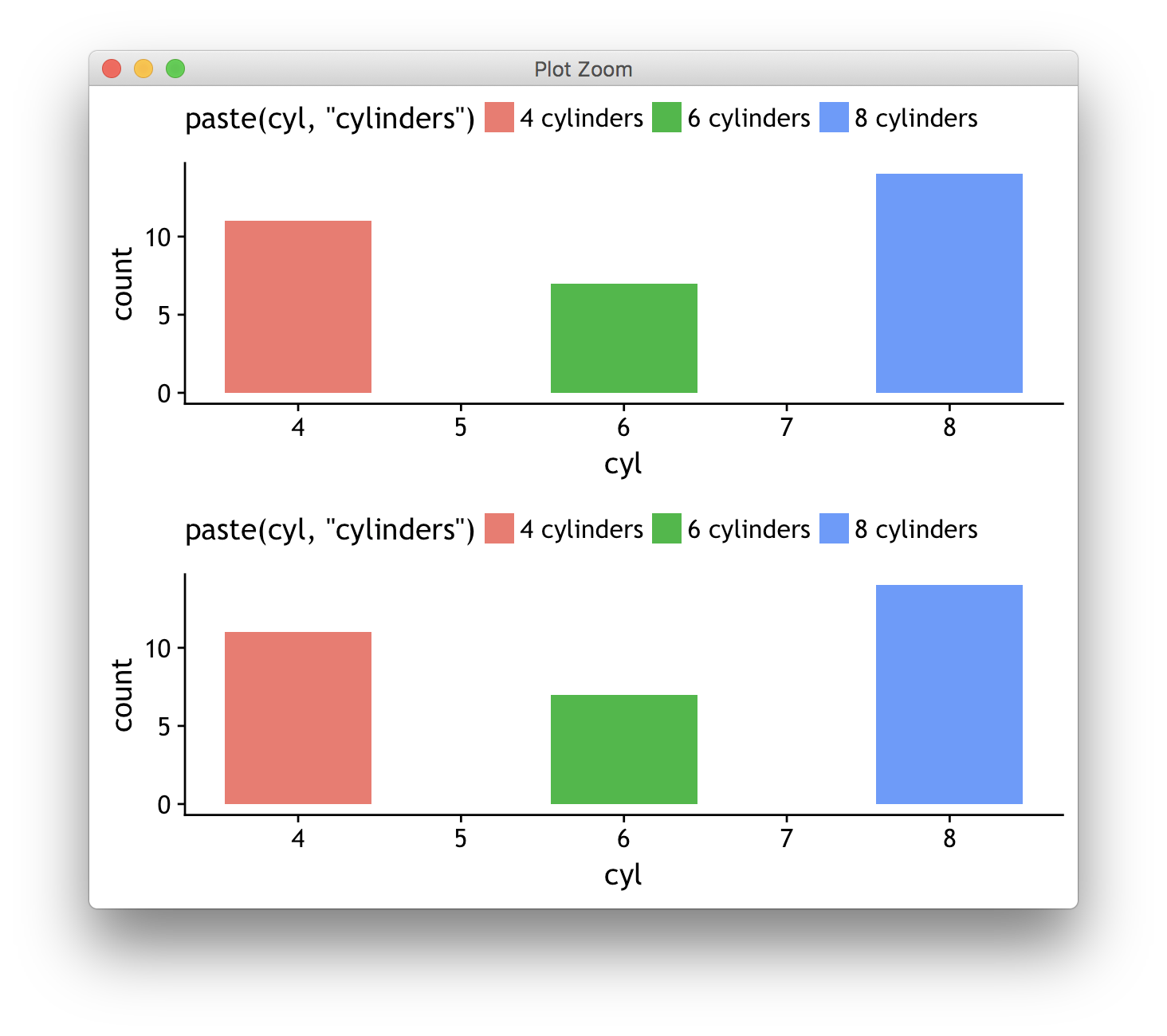Author

steveharoz commented Mar 16, 2018

 I installed the dev version `devtools::install_github("wilkelab/cowplot")` ``````library(Cairo) library(cowplot) plotTemp = ggplot(mtcars) + aes(x=cyl, fill=paste(cyl, "cylinders")) + geom_bar() + theme(text = element_text(family="Comic Sans MS")) # distinctive font `````` The RStudio viewer can't use the correct fonts ``````cowplot::plot_grid(plotTemp, plotTemp, ncol=1, align="v") ``````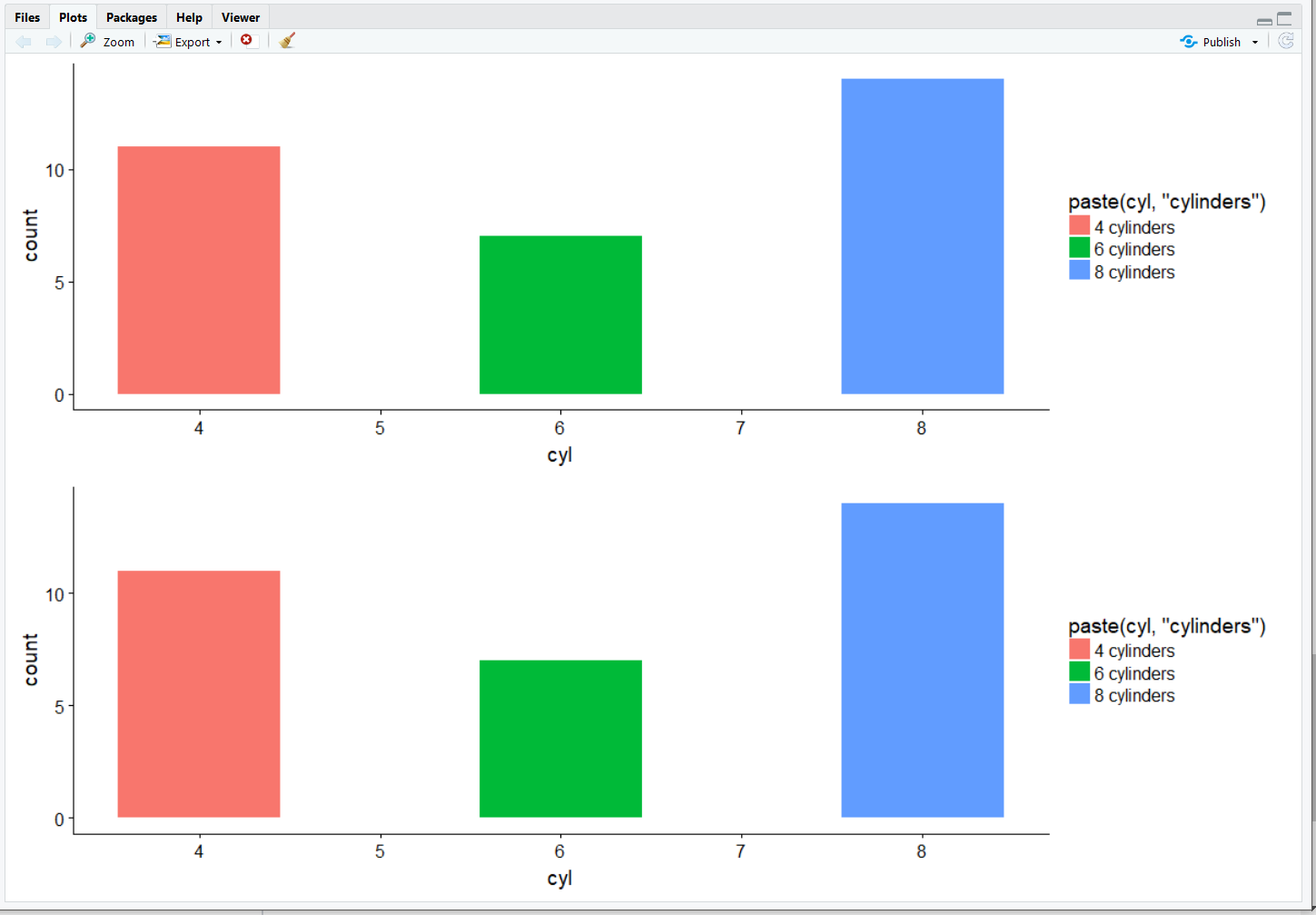``````There were 50 or more warnings (use warnings() to see the first 50) > warnings() Warning messages: 1: In grid.Call(C_textBounds, as.graphicsAnnot(x\$label), ... : font family not found in Windows font database `````` Using the Cairo viewer works! ``````CairoWin() cowplot::plot_grid(plotTemp, plotTemp, ncol=1, align="v") ``````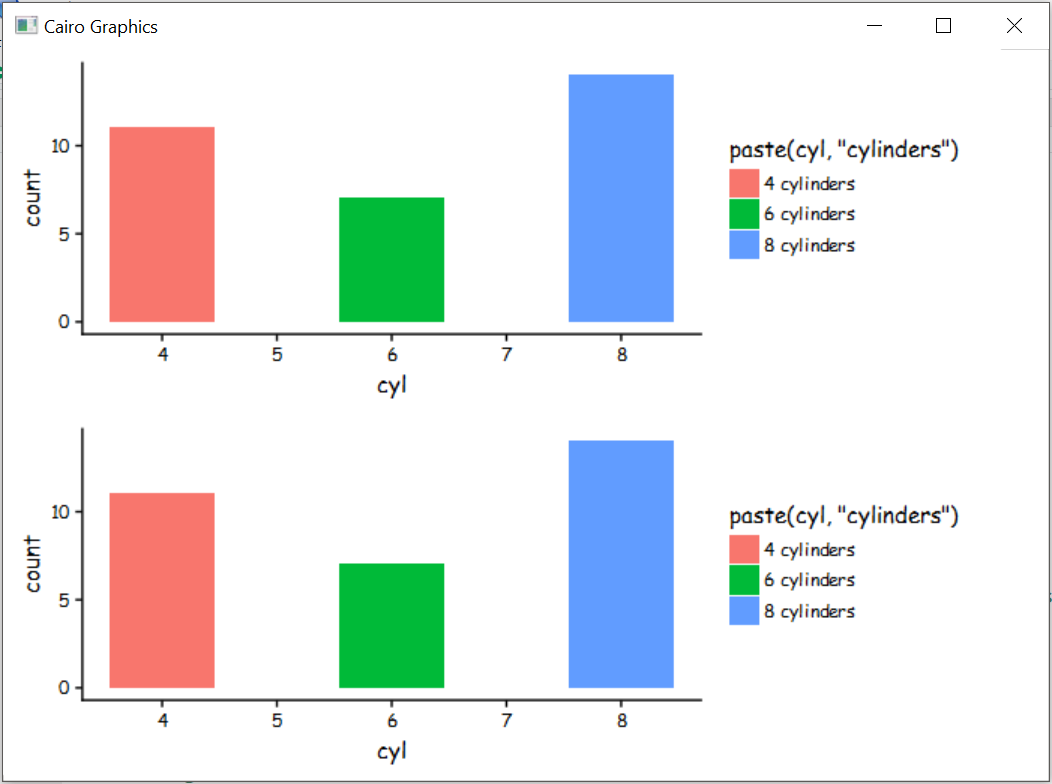``````plotTemp = plotTemp + theme(legend.position = "top") cowplot::plot_grid(plotTemp, plotTemp, ncol=1) ``````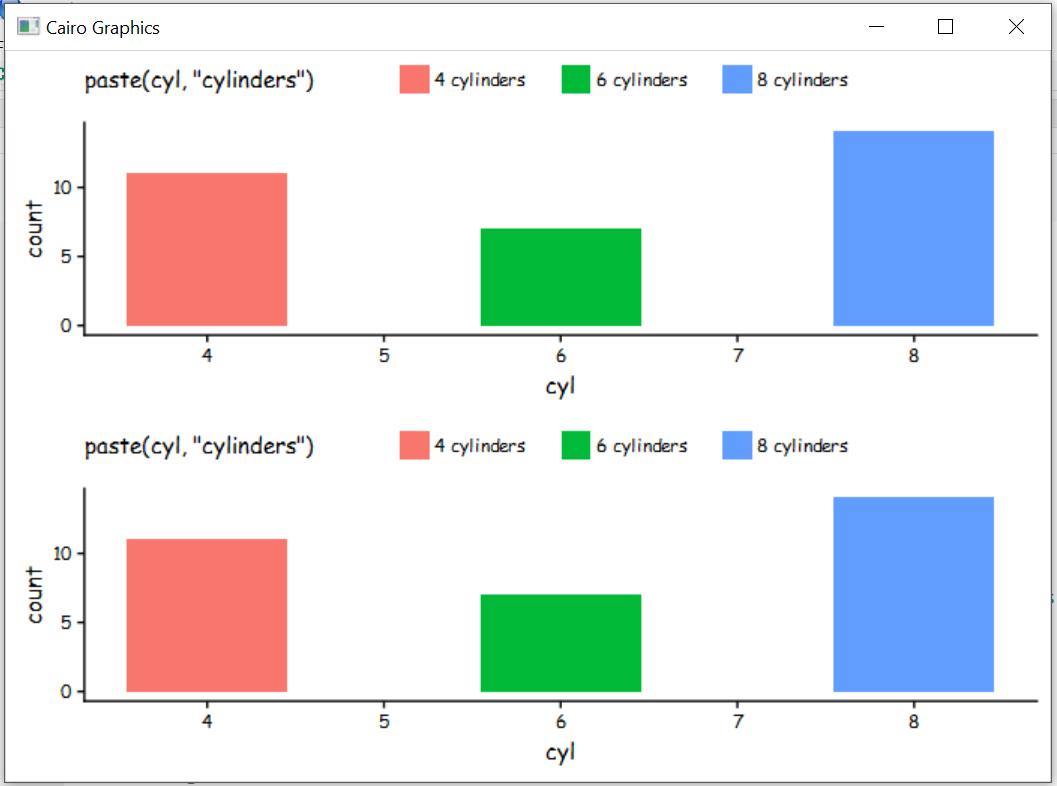Using Cairo to save works too ``````Cairo(1000, 750, 'cowplottest.png', bg = 'white') last_plot() dev.off() `````` So it seems that using cowplot on Windows requires using the Cairo graphics device
Contributor

clauswilke commented Mar 17, 2018

 Thanks. There are still some subtle font metric issues that I need to resolve, but at least it's not blatantly wrong.
Contributor

clauswilke commented Mar 17, 2018 • edited

 I've done some more digging. It turns out there is no single solution that always works. The problem is that we need to open a null graphics device for some of the cowplot operations, and there is no universal null graphics device that works reliably in all circumstances. On OS X, for example, the solution I pushed earlier does not work. (Cairo is broken there. It ignores font settings.) So, the solution is to allow the user to choose their own null device. This means you can always choose the one that works the best in your situation. Below, I show how the various null devices work in OS X, and why Cairo does not work. ``````library(cowplot) # make plot with narrow font and without margins p <- ggplot(iris, aes(Sepal.Length, Sepal.Width, color = Species)) + geom_point() + theme_minimal(base_family = "Arial Narrow") + theme(legend.margin = margin(0, 0, 0, 0), plot.margin = margin(0, 0, 0, 0)) # pdf null device (the default) without extrafont doesn't work ggdraw(p) ## There were 18 warnings (use warnings() to see them) ``````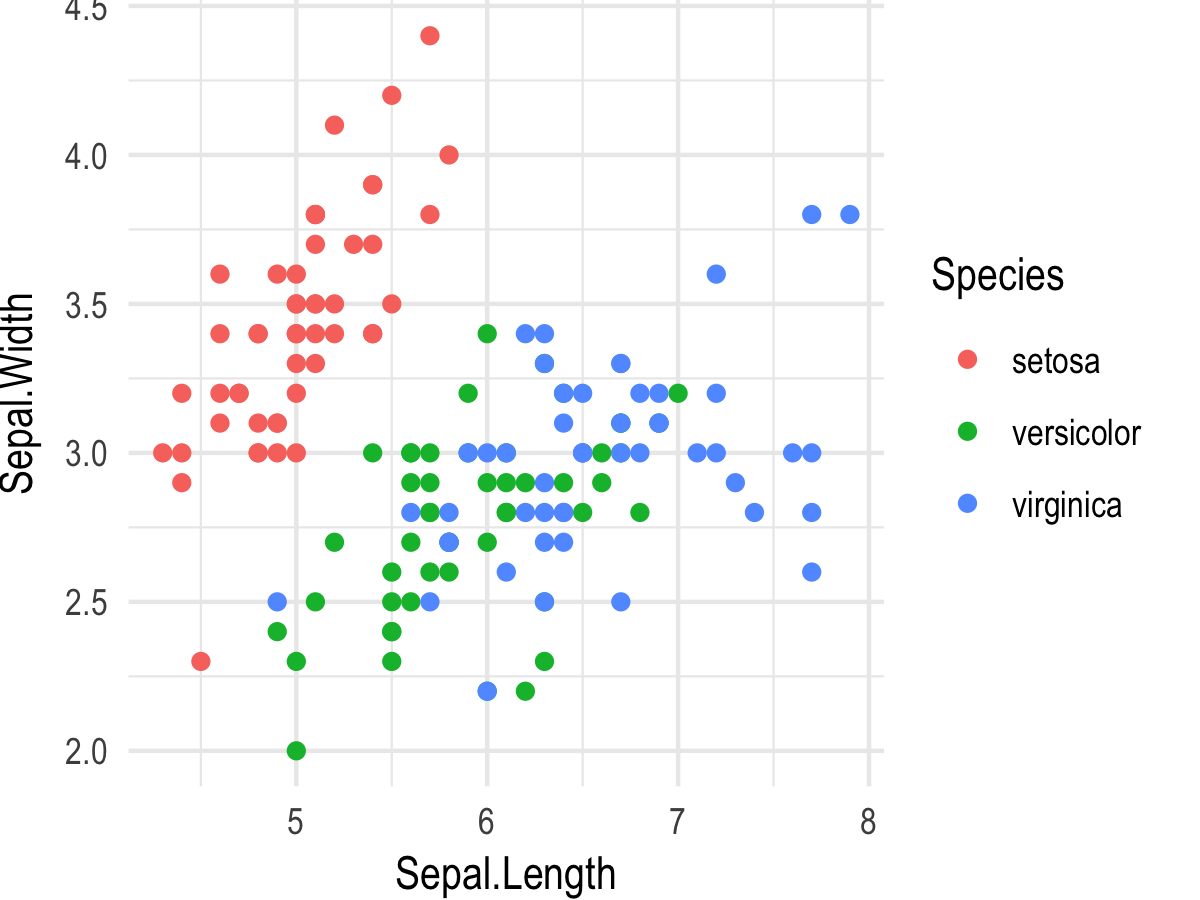We see that things don't work properly because (i) the code generates 18 warnings (about missing fonts) and (ii) the legend text doesn't extend all the way to the end of the image. ``````# cairo null device gets font metrics wrong set_null_device("cairo") ggdraw(p) ``````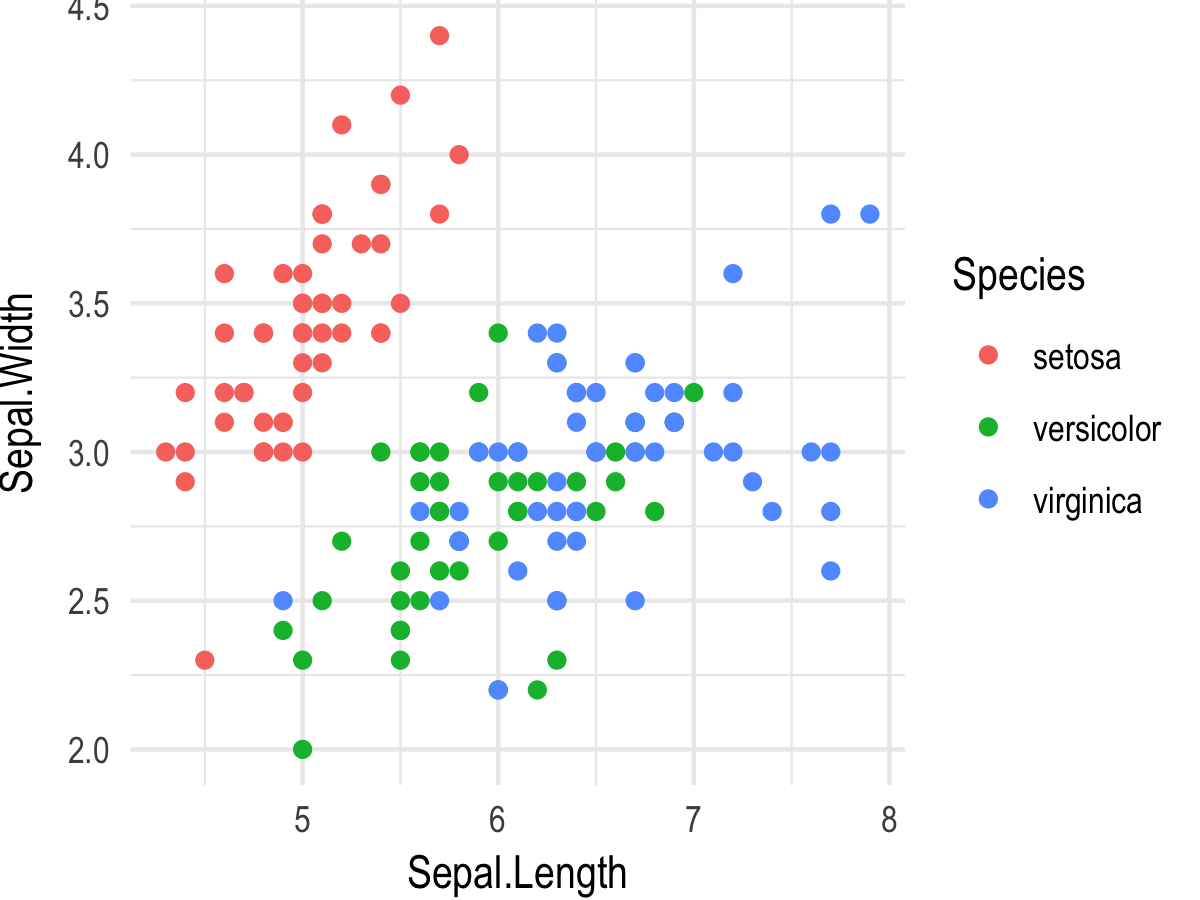Same issue as before, but without warnings. Cairo simply ignores all font settings. ``````# png null device works without problems set_null_device("png") ggdraw(p) ``````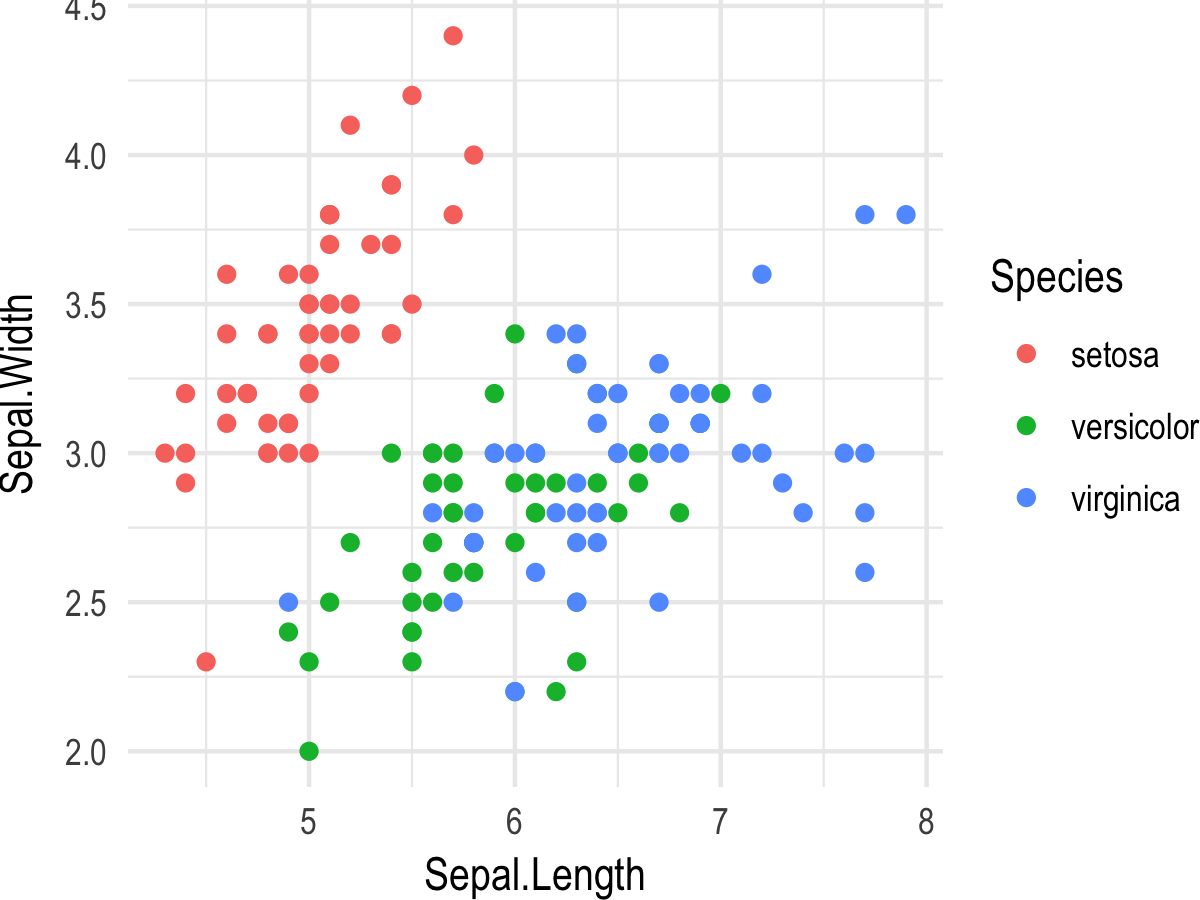``````# on OS X, pdf null device with extrafont works library(extrafont) set_null_device("pdf") ggdraw(p) ````````````# on OS X, jpeg null device also works jpeg_null_device <- function() {jpeg(filename = "jpeg_null_plot.jpg")} set_null_device(jpeg_null_device) ggdraw(p) ``````Author

steveharoz commented Mar 17, 2018

 Just updated. It's behaving differently on my windows computer. ``````library(cowplot) # make plot with specific font and without margins p <- ggplot(iris, aes(Sepal.Length, Sepal.Width, color = Species)) + geom_point() + theme_minimal(base_size = 20, base_family = "Comic Sans MS") + theme(legend.margin = margin(0, 0, 0, 0), plot.margin = margin(0, 0, 0, 0)) `````` ``````# pdf null device (the default) without extrafont doesn't work ggdraw(p) ## There were 20 warnings (use warnings() to see them) `````` ``````# Note that cairo null device gives me warnings (unlike on your computer) set_null_device("cairo") ggdraw(p) ## Warning messages: ## 1: In grid.Call(C_textBounds, as.graphicsAnnot(x\$label), x\$x, x\$y, : ## font family not found in Windows font database `````` ``````# extrafont and cairo null device works! library(extrafont) ggdraw(p) ggdraw(p + theme(legend.position = "top")) `````` ``````# pdf null device messes up borders set_null_device("pdf") ggdraw(p) ``````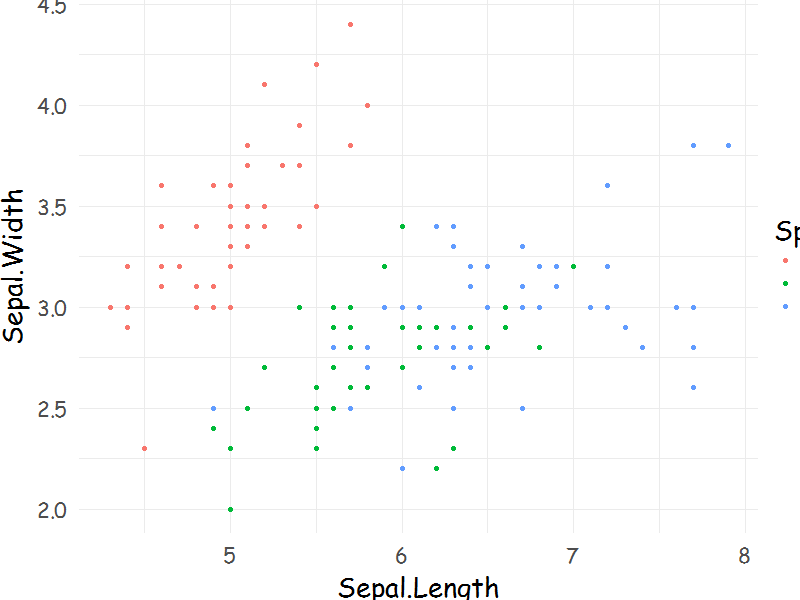Open

Contributor

clauswilke commented May 5, 2018

 Closing this issue. As far as I can tell, this works fine now. I have been using non-standard fonts for several months without problems.